# Case study AharonovBom effect J Planelles Case study

• Slides: 18Case study: Aharonov-Bom effect J. PlanellesCase study: effects on the energy spectrum of a beam of magnetic flux restricted to a small area inside, outside and piercing a quantum ring.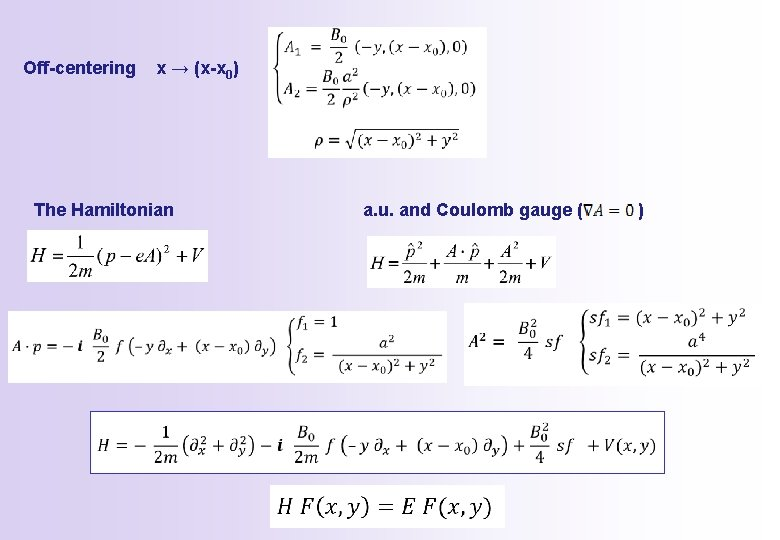Off-centering x → (x-x 0) The Hamiltonian a. u. and Coulomb gauge ( )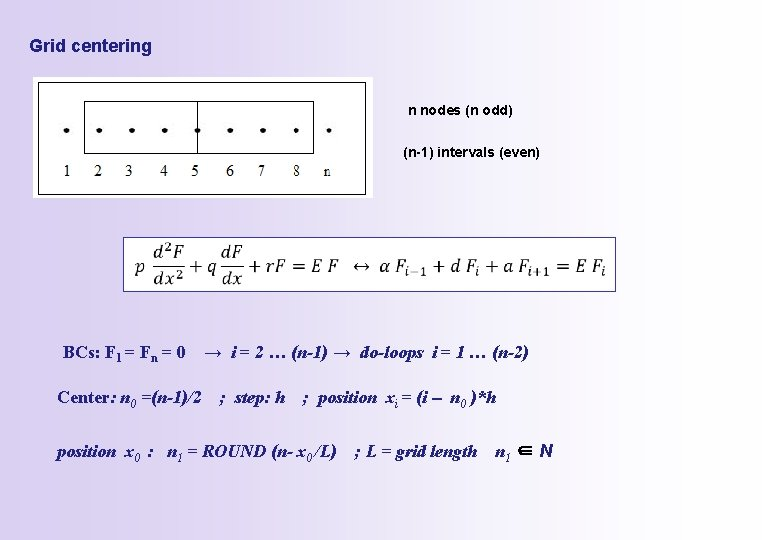Grid centering n nodes (n odd) (n-1) intervals (even) BCs: F 1 = Fn = 0 Center: n 0 =(n-1)/2 → i = 2 … (n-1) → do-loops i = 1 … (n-2) ; step: h ; position xi = (i - n 0 )*h position x 0 : n 1 = ROUND (n- x 0 /L) ; L = grid length n 1 ∈ NLinear System of equations BCs: F 1, j = Fn, j = Fi, 1 =Fi, n = 0i=2 i=3 i = n-1 a d a b b j=2 F 1, 2 = 0 F 2, 2 F 3, 2 F 2, 1 = 0 F 2, 3 j=3 F 1, 3= 0 F 2, 3 F 3, 3 F 2, 2 F 2, 4 . . . . j=n-1 F 1, (n-1)= 0 F 2, (n-1) F 3, (n-1) F 2, (n-2) F 2, n= 0 j=2 F 2, 2 F 3, 2 F 4, 2 F 3, 1 = 0 F 3, 3 j=3 F 2, 3 F 3, 3 F 4, 3 F 3, 2 F 3, 4 . . . . j=n-1 F 2, (n-1) F 3, (n-1) F 4, (n-1) F 3, (n-2) F 3, n= 0 . . . . j=2 F(n-2), 2 F(n-1), 2 Fn, 2= 0 F(n-1), 1 = 0 F(n-1), 3 j=3 F(n-2), 3 F(n-1), 3 Fn, 3= 0 F(n-1), 2 F(n-1), 4 . . . . j=n-1 F(n-2), (n-1) F(n-1), (n-1) Fn, (n-1) = 0 F(n-1), (n-2) F(n-1), n= 0a b d b a 0 0 0 0 0 0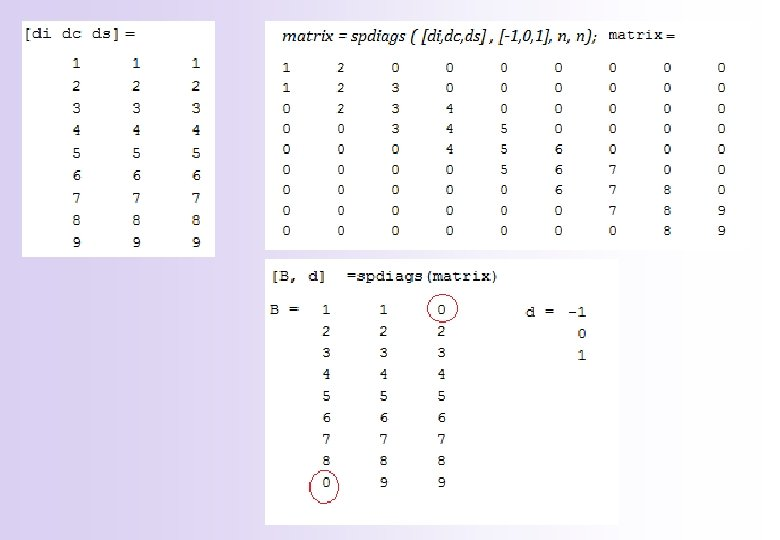Code matlab/octave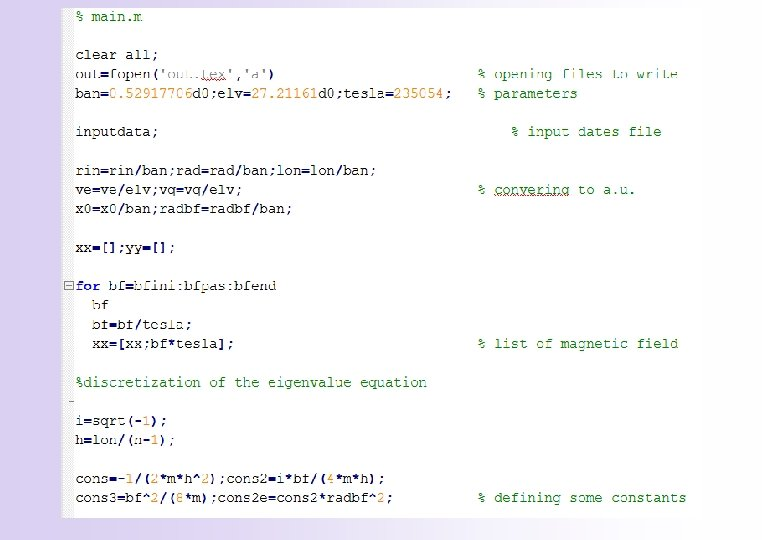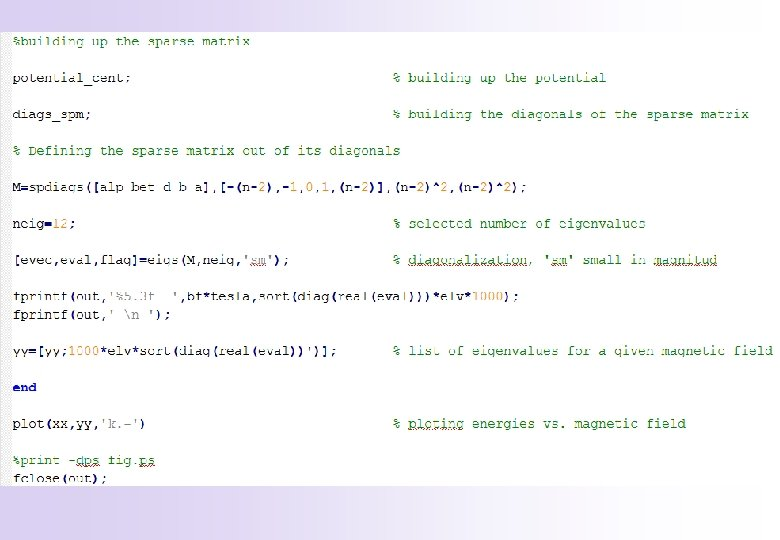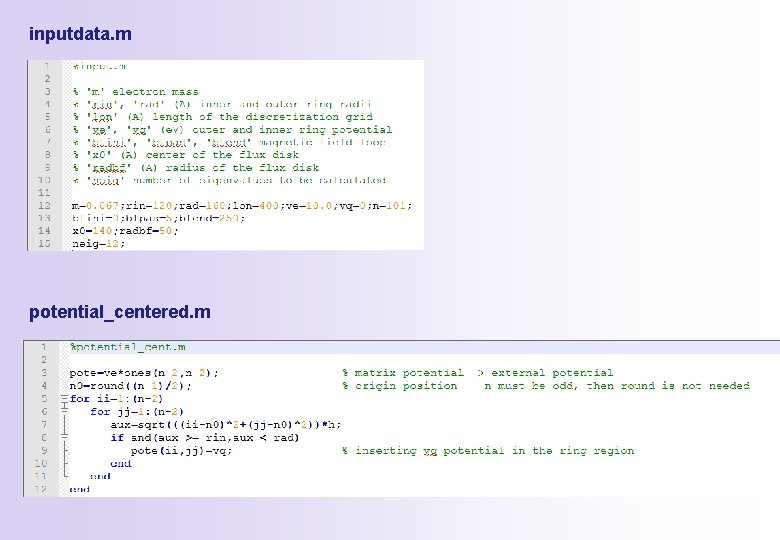inputdata. m potential_centered. m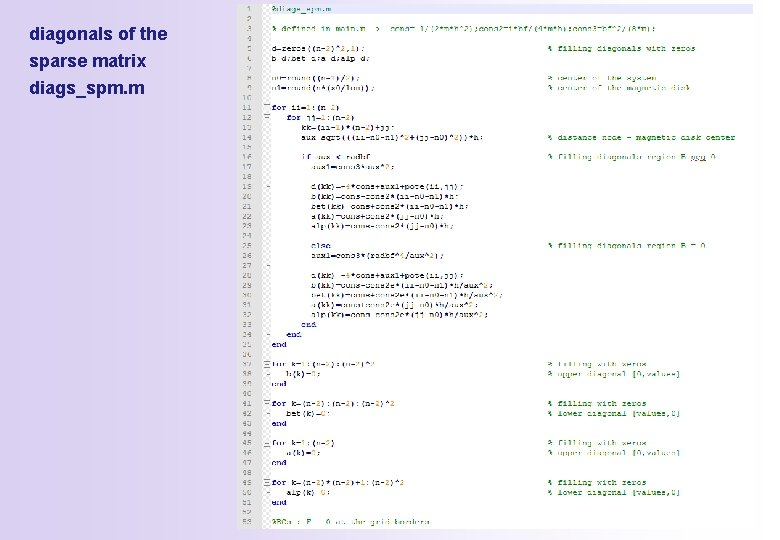diagonals of the sparse matrix diags_spm. m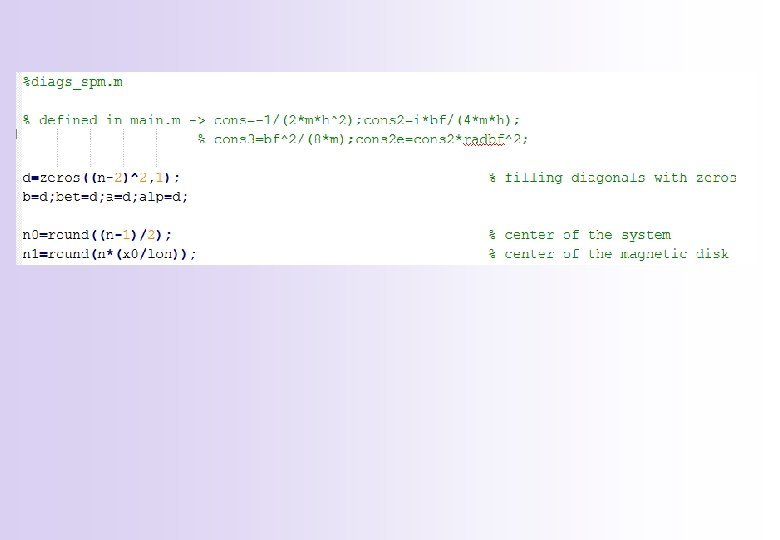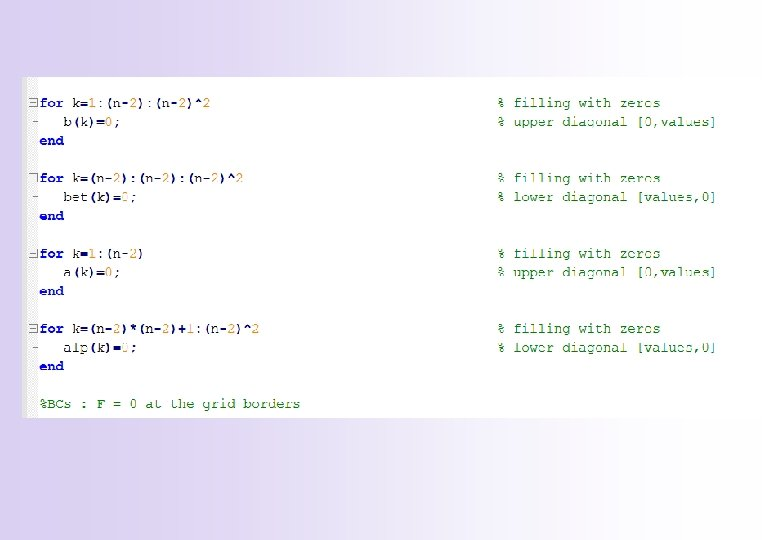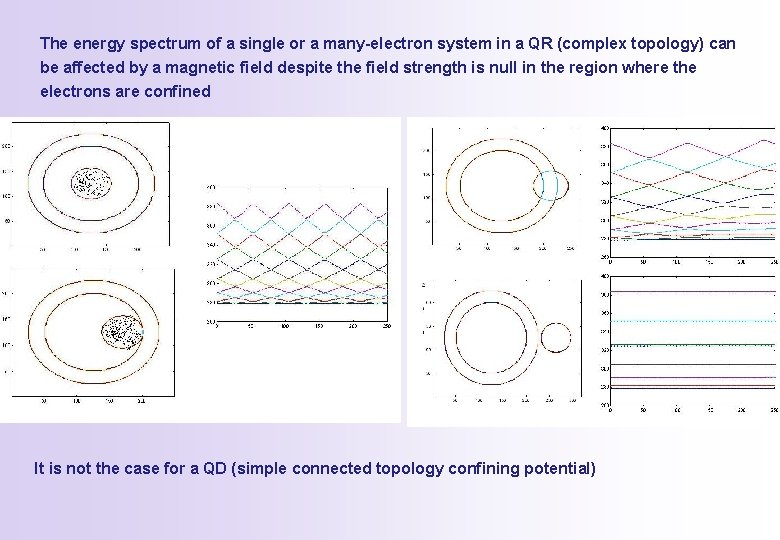The energy spectrum of a single or a many-electron system in a QR (complex topology) can be affected by a magnetic field despite the field strength is null in the region where the electrons are confined It is not the case for a QD (simple connected topology confining potential)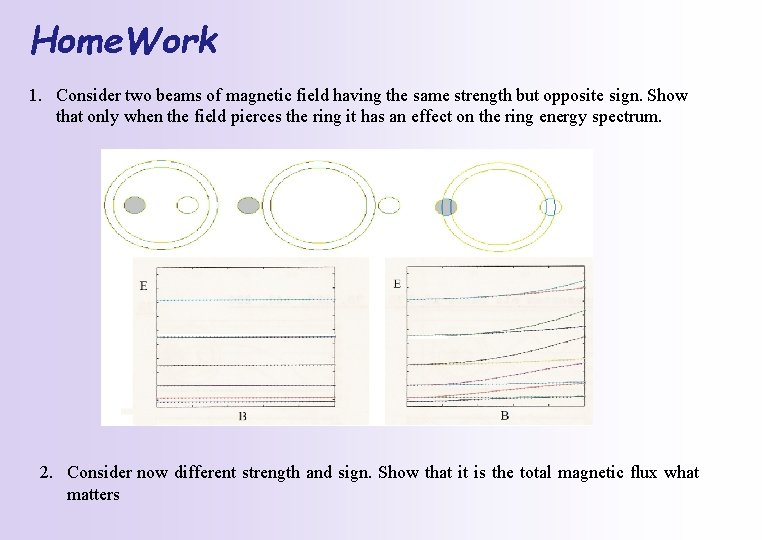Home. Work 1. Consider two beams of magnetic field having the same strength but opposite sign. Show that only when the field pierces the ring it has an effect on the ring energy spectrum. 2. Consider now different strength and sign. Show that it is the total magnetic flux what matters